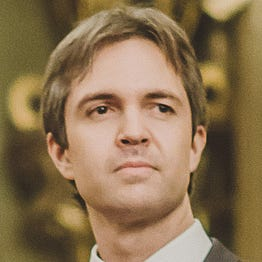# Quantum Theory and Knots: Exploring Fascinating Links Between Apparently Disparate Domains

## How Quantum Theories Impacted Mathematics, Linking Different Domains, Revolutionizing Entire Subfields and Becoming a Consistent Source of Exciting New Problems

The purpose of this article is to provide a comprehensive account of the profound and unexpected relationship, discovered in the 1980s, between a type of quantum theory and the mathematical theory of knots, a subject belonging to the field of topology. Topology studies geometric objects which are preserved under continuous deformations (such as stretching, twisting, and bending). An example of such transformation is shown below. In the illustration, a mug is continuously deformed into a donut.

# The Power of Dualities

## A Brief Summary with Examples

Dualities are equivalences between distinct descriptions (or formulations) of the same physical system. These descriptions are frequently referred to as “duality frames” and are often complementary to each other.

As remarked in my first article about dualities (see link below), their significance for developing contemporary fundamental physics cannot be overstated.

# A Simple Case of Duality

The following trivial example, borrowed from Vafa (1998), illustrates the basic idea. On a two-dimensional space (say, the real plane), if a geometrical property is true, it is also true for its reflection.

# Why The Most Powerful Idea You’ve Never Heard of May Require a Radical Reformulation of the Laws of Physics

## How a Concept Known as “Duality” Reshapes Our View of the World, Provides Insights into Previously Inscrutable Areas of Physics, and Unexpectedly Unifies Disparate Branches of Contemporary Mathematics

One of the most profound insights of modern physics is called duality. Dualities were at the core of several breakthroughs in fundamental physics, considerably changing how physicists think about fundamental principles. Moreover, they provided unexpected mathematical results that are likely to help developing several branches of that subject, possibly for decades.

They also have identified completely unforeseen connections, thus eliminating previously hidden redundancies in physical theories. In the words of Joseph Polchinski (1954–2018), one of the preeminent physicists of the past 50 years (see link), “the existence of dualities points to a great unity in the structure of theoretical physics.”

# Aspects of Topological Quantum Field Theory

## How to Show That the Famous Jones Polynomial Invariant Has Its Origin in Physics

This article will provide a detailed account of Witten’s derivation, based on the Chern-Simons theory, of the skein relation obeyed by the Jones polynomials (in my last article, linked below, I provided only the rationale underlying the derivation).

But first, let me first provide a quick review of topological quantum field theories, which were explored in great detail in my previous article.

## Important Information

I considerably expanded the content of this article, and the new, improved version was joined to my previous one. …

# Deriving Einstein’s Gravity Equations From Thermodynamics

## Is Gravity Just an Average of the Behavior of Unknown “Atoms” of Spacetime?

Emergent gravity is an idea in quantum gravity according to which the fabric of spacetime is not fundamental but emerges as a coarse-graining approximation of underlying (still unknown) microscopic degrees of freedom (similarly to a gas emerging from a large sampling of atoms or molecules). In the words of Huggett and Wuthrich, emergent gravity is the view that gravity arises due to the “collective action of the dynamics of more fundamental non-gravitational degrees of freedom.”

# How Richard Feynman Reinvented Quantum Theory

## The Space-Time Approach to Quantum Mechanics

The American theoretical physicist Richard Feynman is one of the best-known scientists in the world (see this link). Feynman made several contributions to theoretical physics. These include: a new formulation of quantum mechanics using path integrals, the theory of quantum electrodynamics (including the development of his famous pictorial representations known as Feynman diagrams), his contributions to the explanation of the phenomenon of superfluidity of supercooled liquid helium, and his pioneering work in quantum computing and nanotechnology. In 1965, he was one of the recipients of the Nobel Prize in Physics.

# Quantum Tunneling, Bubble Universes and the End of the World

## The Catastrophic Consequences of Living in a Universe with a False Vacuum

A vacuum is a space with as little energy in it as possible. However, a vacuum is not entirely empty. It contains quantum fields. Quantum fields are obtained after the quantization of classical fields, which are functions of spacetime coordinates (such as, for example, the electromagnetic field). Mathematically quantum fields are operator-valued functions of space and time.

# Why Time Is Encoded in the Geometry of Space

## An Introduction to Geometrodynamics

In Albert Einstein’s original formulation of general relativity (his theory of gravity), the fundamental field is the metric tensor g and the theory is covariant in spacetime. Covariance (more precisely general covariance) “consists of the invariance of the form of physical laws under arbitrary coordinate transformations.” The idea is that since coordinates are only human-made labels, physical laws shouldn’t depend on the way they are chosen.

In general relativity, the action (“an attribute of the dynamics of a system from which its equations of motion can be derived”) is called Einstein–Hilbert action:

# A Gentle Explanation of E= mc²

## Deriving Einstein’s Famous Mass-Energy Equivalence Formula

The mass-energy equivalence formula E = mc² defines a relationship between the mass m and the energy E of a body in its rest frame (the rest frame is the frame of reference where the body is at rest). The square of the speed of light is an enormous number, and therefore a small amount of rest mass is associated with a tremendous amount of energy.

# Length Contraction in Einstein’s Theory of Relativity

## Why Moving Objects are Shortened